## Orthogonal Projection of a Straight Line

If a straight line p is not a ray of projection (not perpendicular to either projection plane), then its horizontal and vertical projections are lines p' and p''.
Hence, in Monge's projection, a straight line is represented by a pair of projections (p',p'').

A point T lies on a line p if its horizontal projection lies on the horizontal projection of the line and its vertical projection lies on the vertical projection of the line.

The reverse also holds, so we can state: Tp <=> T'p' & T''p''.

A point P1 where the line p intersects the horizontal plane is called horizontal trace of the line p, P1 = pΠ1.
A point P2 where the line p pierces the vertical plane is called the vertical trace of the line p, P2 = pΠ2.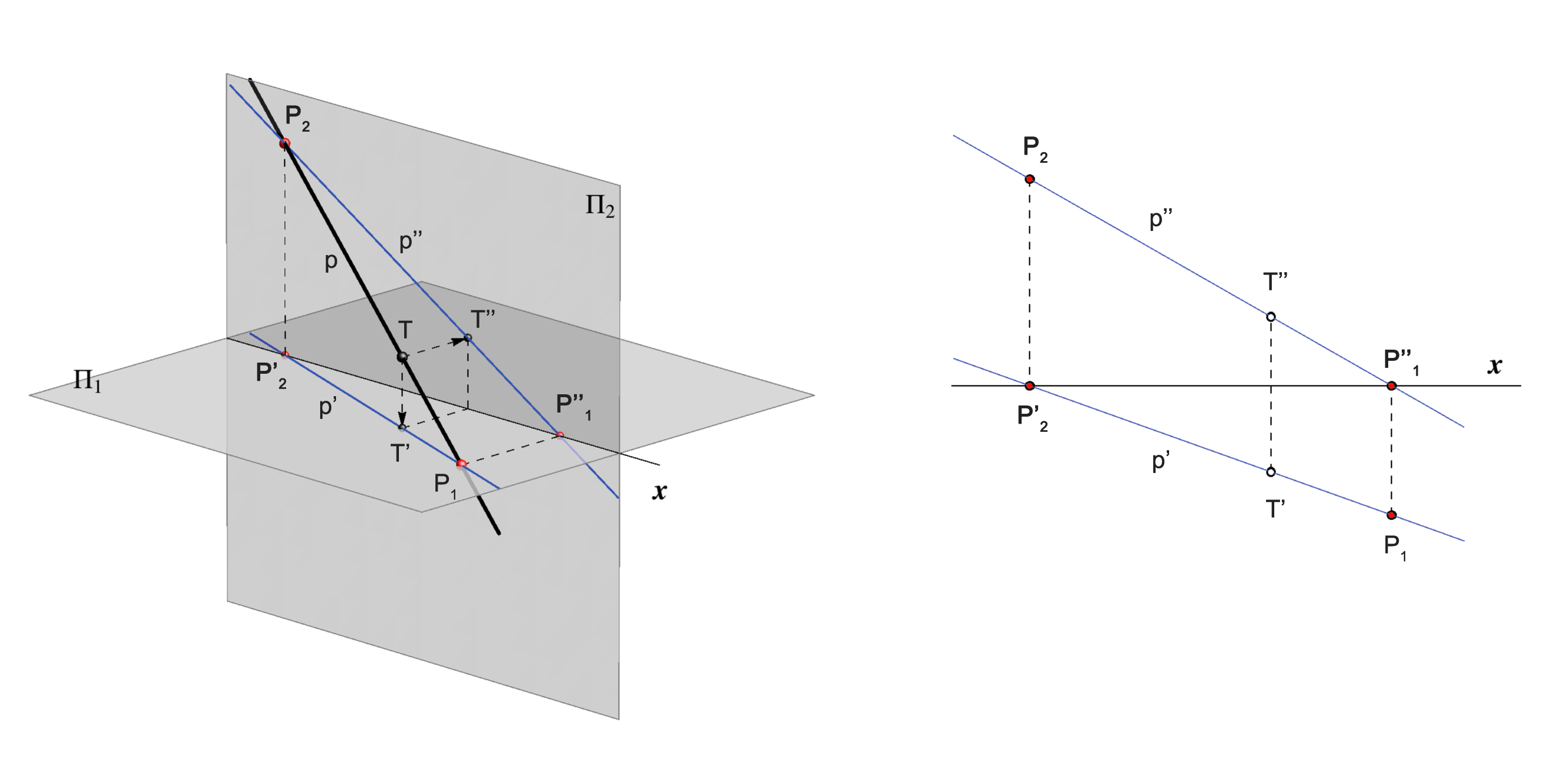CONSTRUCTION of TRACES

### Angles of inclination of a straight line to the planes of projection

 First angle of inclination  ω1 of a line p is the angle between the line and the horizontal plane Π1. It is also called slope angle. It equals the angle between the line and its horizontal projection. ω1 = ∠(p,p'). Second angle of inclination ω2 of a line p is the angle between the line and the vertical plane Π2, i.e. ω2 = ∠(p,p''). Construction of these angles will be explained later.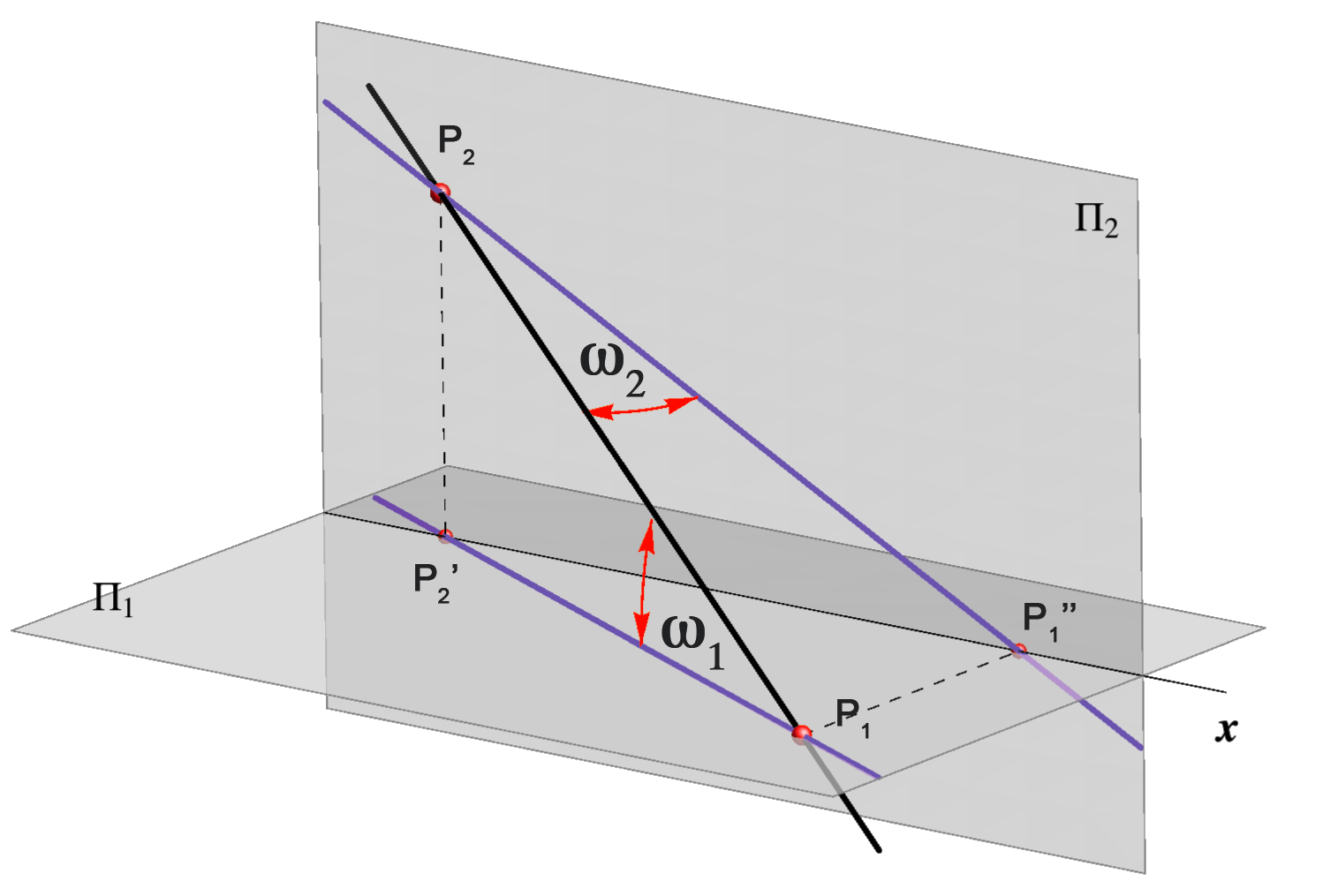### Straight lines in special positions

• A line parallel to the horizontal plane Π1 is called horizontal (level) line and all its points have the same distance to the horizontal plane, i.e. have the same z-coordinate.
Therefore, the vertical projection of that line is parallel to the ground line x. The reverse also holds, so we can state: p || Π1 <=> p'' || x.
A horizontal line does not have a horizontal trace.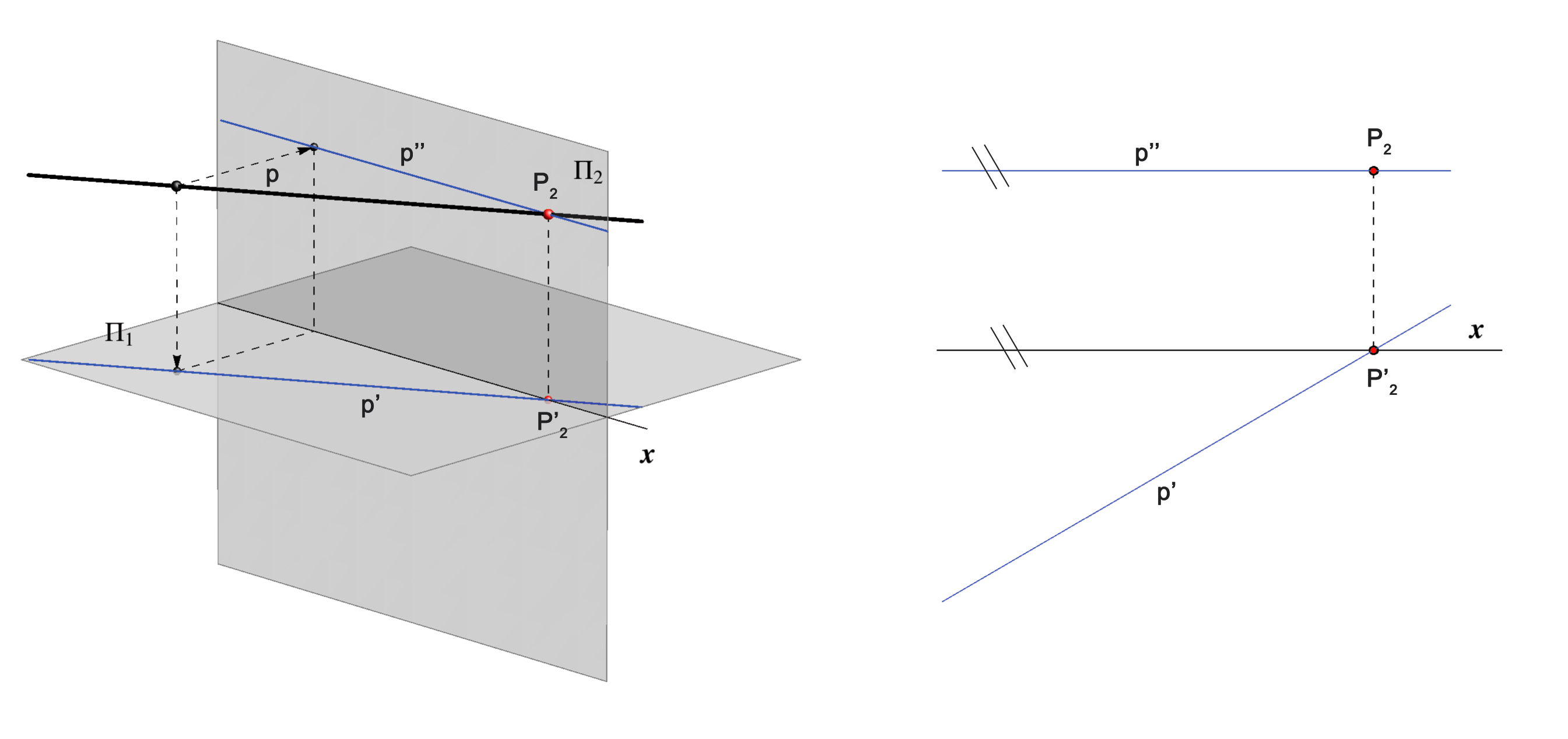If the vertical projection of a line is parallel to the x-axis, then the line is parallel to the horizontal plane.

• If a line is parallel to the vertical plane Π2 all its points have the same distance to the vertical plane, i.e. have the same y-coordinate. Such line is called vertical (frontal) line. Hence, the horizontal projection of the line is parallel to the x-axis. The reverse also holds, so we state:  p || Π2 <=> p' || x.
A vertical line does not have a vertical traces.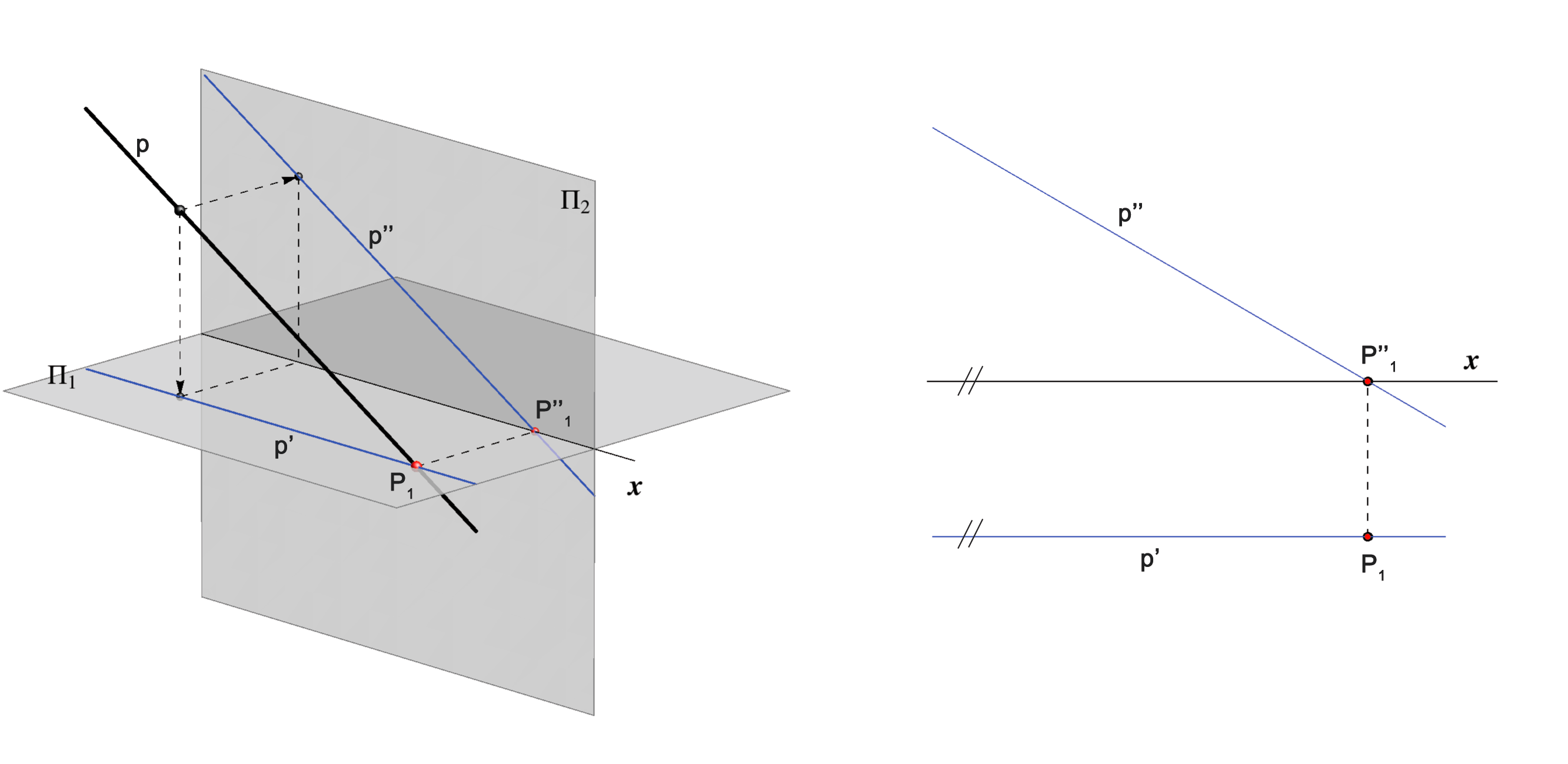If the horizontal projection of a line is parallel to the x-axis, then the line is parallel to the vertical plane.

• If a line is contained  in the plane Π1 ( Π2), then the vertical (horizontal) projection of the line coincide with x-axis. The reverse holds.

• If a line is parallel to the x-axis, then its horizontal and vertical projections are also parallel to x. The reverse holds.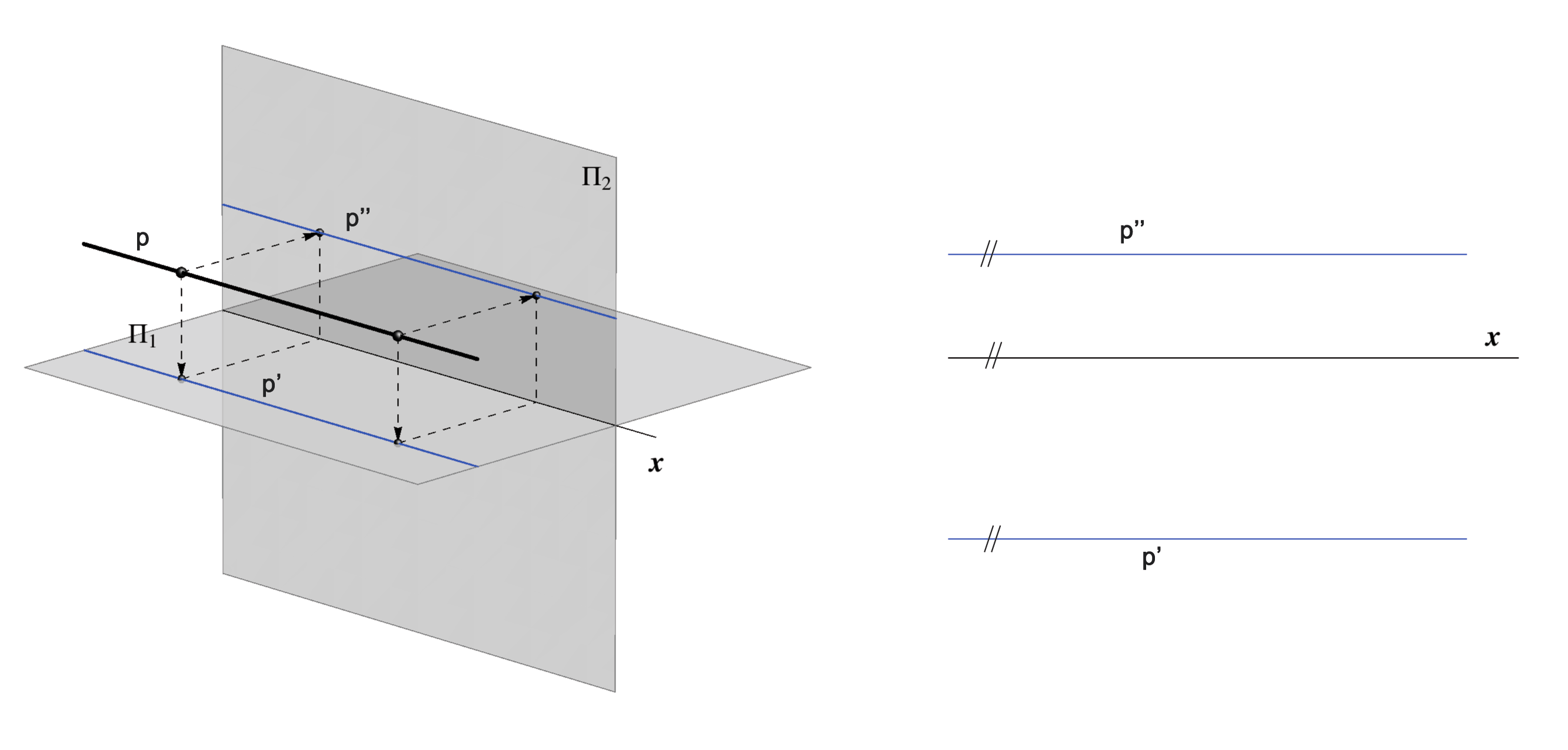If the horizontal and vertical projections of a line are parallel to the x-axis, then the line is parallel to x.

• If a line is perpendicular  to the plane Π1 , then its horizontal projection is a  point and its vertical projection is  perpendicular to the x-axis
• If a line is perpendicular  to the plane Π2 , then its vertical projection is a  point and its horizontal projection is  perpendicular to x-axis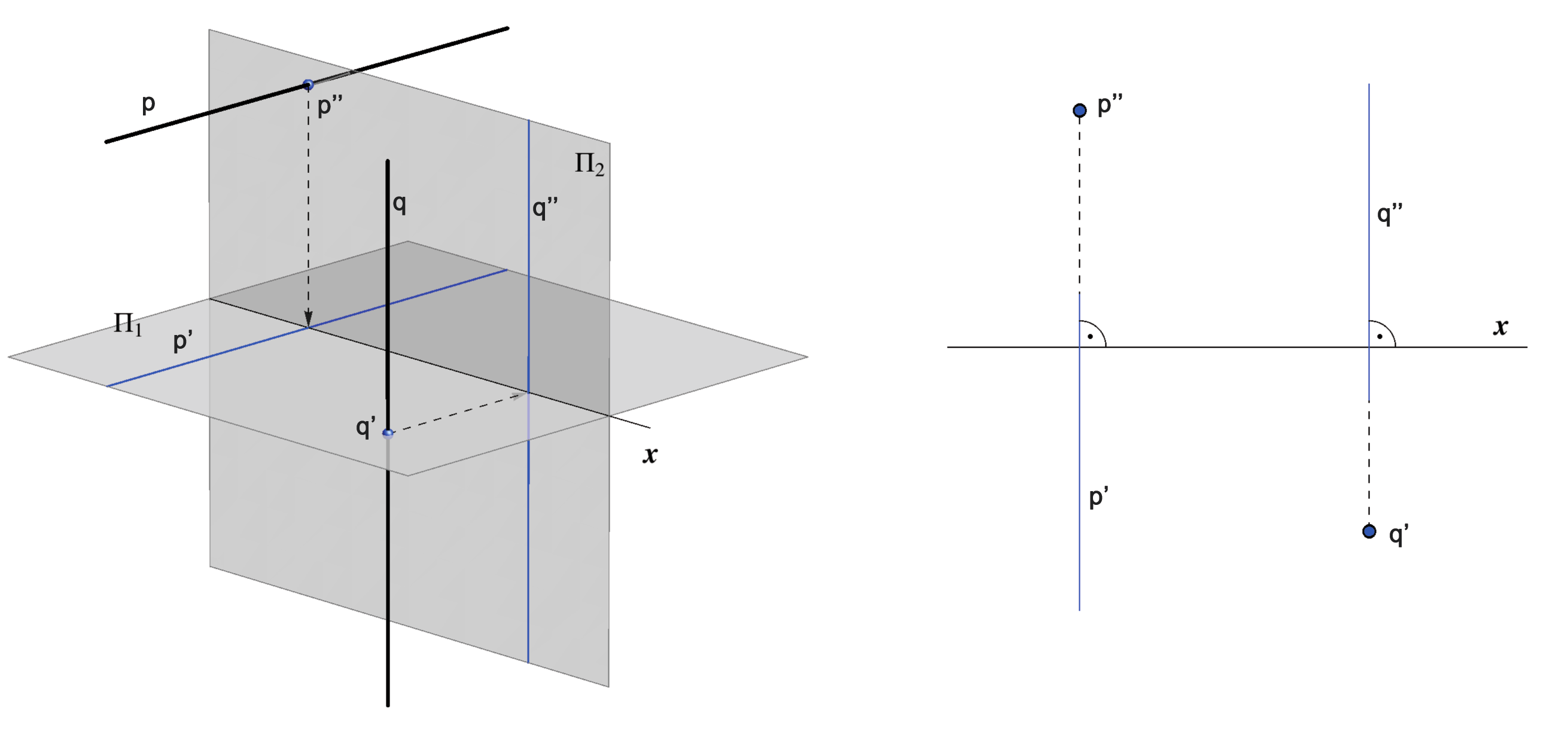A line perpendicular to either projection plane is a ray of projection and projects to a point.

### Relative positions of lines

• If the lines a andare parallel, then they have parallel horizontal projections and parallel vertical projections. The reverse also holds, so we can state: a || b <=> a' || b' & a'' || b''.

• If the lines a and b are intersecting lines, then the intersection of their  horizontal projections and the intersection of their vertical projections lie on the same line of recall. The reverse holds.

• If the lines a and b are skew lines, then the intersections of their horizontal and vertical projections do not lie on the same line of recall. The reverse holds.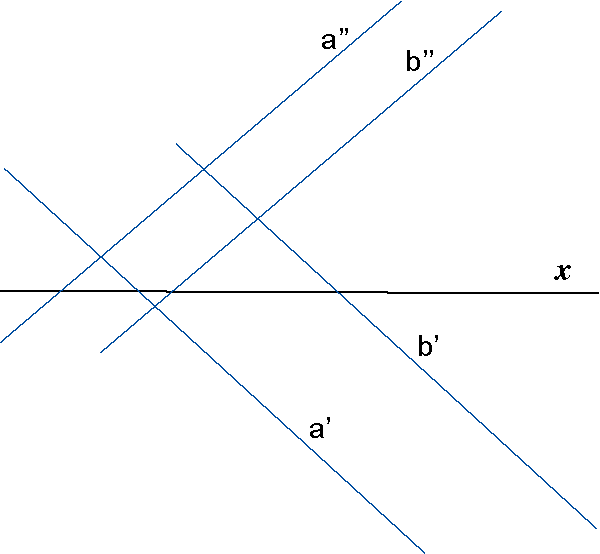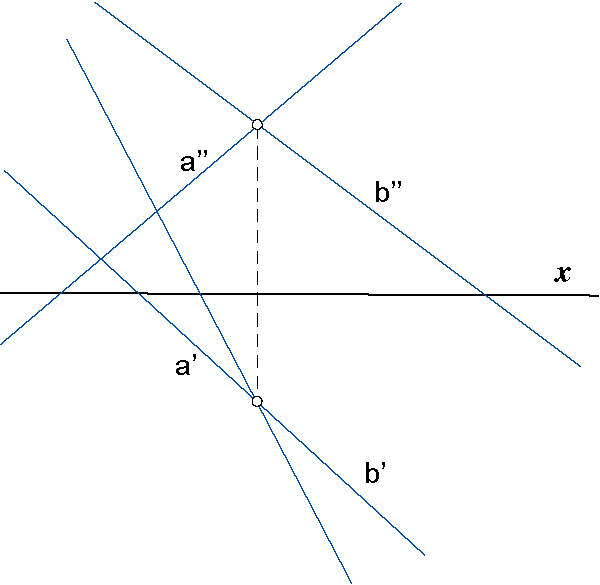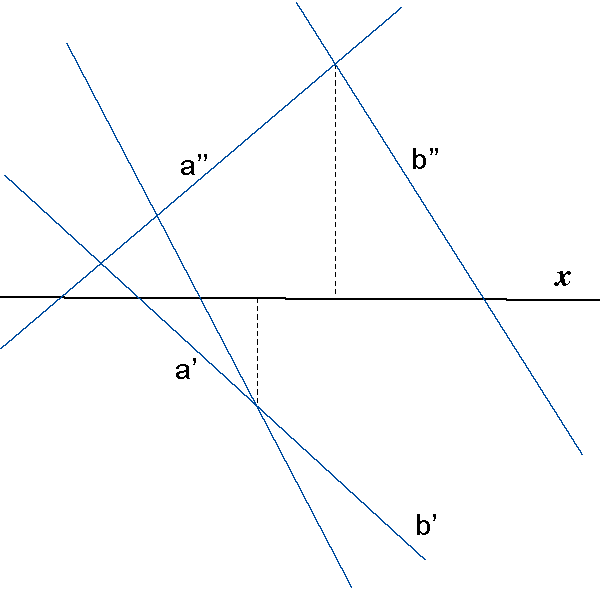Parallel lines Intersecting lines Skew lines

### Straight line, symmetry plane, coincidence plane

Due to the property of the points lying in the symmetry plane or the coincidence plane, it is very easy to construct the intersection of a line p with these planes.
The construction is shown on the figure below.

• If a line is contained in the symmetry plane, then its horizontal and vertical projections are symmetrical with respect to the x-axis.
• If a line is parallel to the symmetry plane, then its projections have the same angle to the  x-axis.
• If a line is contained in the coincidence plane, then its horizontal and vertical projections coincide.
• If a line is parallel to the coincidence plane, then its projections are parallel.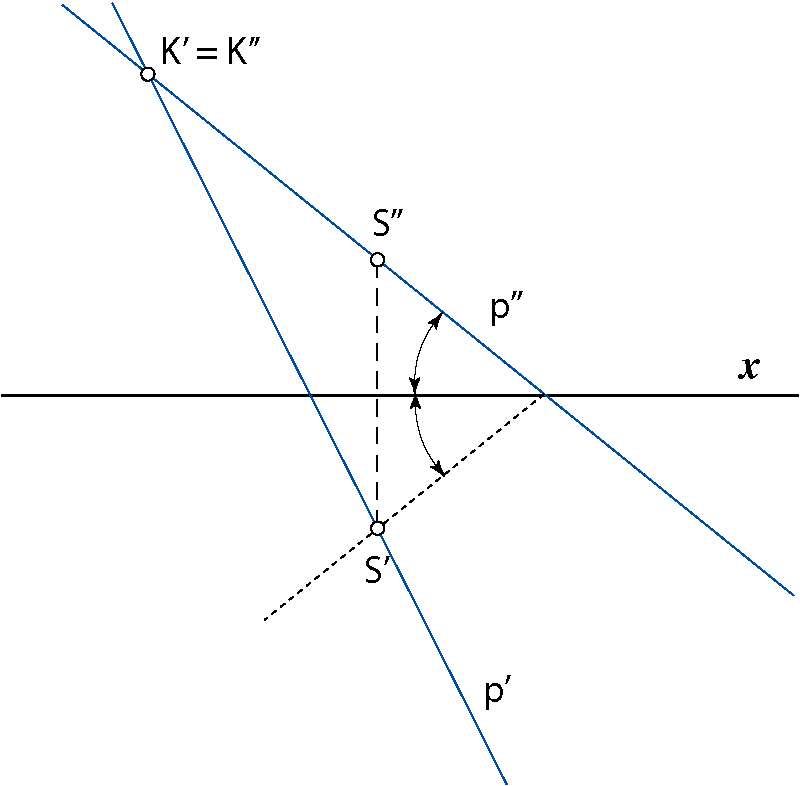S = p ∩ Σ, K = p ∩ Κ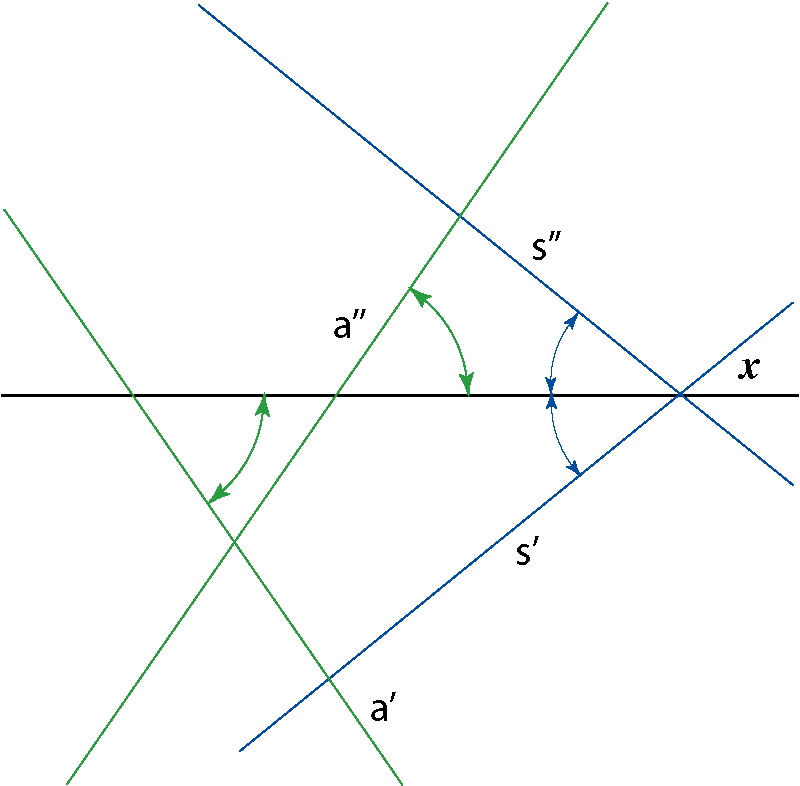s ⊂ Σ, a || Σ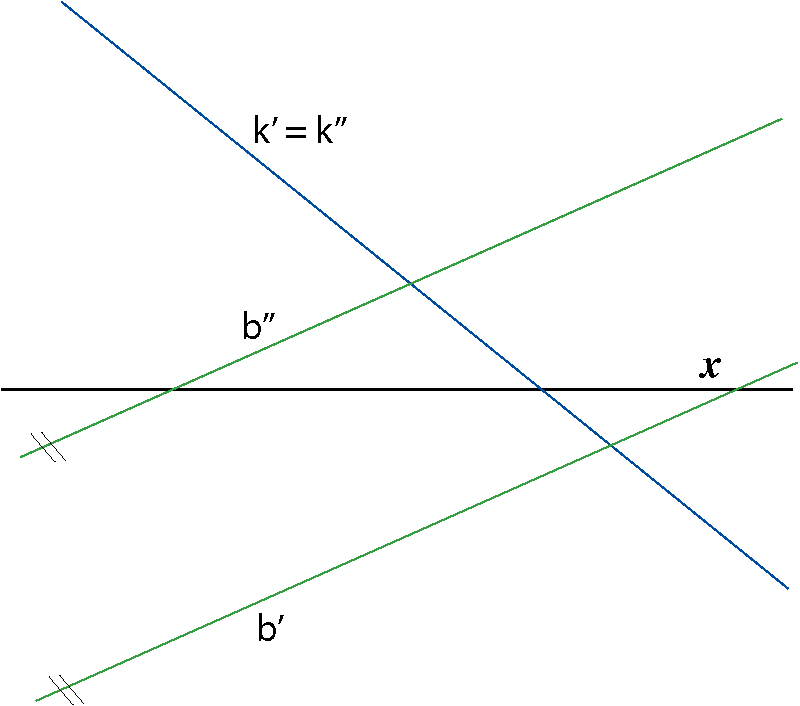k ⊂ Κ, b || Κ

Created by Sonja Gorjanc 3DGeomTeh - Developing project of the University of Zagreb.
Translated by Helena Halas and Iva Kodrnja.